﻿ Geometric Means# Geometric Mean

 Recently, I wrote about Compound Interest and how the Geometric Mean of multiple interest rates can be used as an ‘average’ rate over multiple time periods. Recapping, to calculate a Geometric Mean, we take the nth root of the product of each of the terms.## Theory and Practice

Coincidentally, this week, I needed to calculate geometric means as part of some SQL queries I was running. There were a couple of conceivable problems in the implementation of this:

1. Potential for overflow

2. No aggregate function in SQL

### OverflowMultiplying numbers can result in the product getting big fast. If you don’t know the number of items in your list (and/or their ranges), this can be a worry. Even if you do know the range and scope there are still potential issues with type conversions (implicit or explicit) and potential loss of precision.

### No aggregate function in SQL

SQL is very powerful and has built in functions that take data from multiple rows and aggregates them into a single column. If you’ve written any SQL you’ll have been sure to use some/all of these at various times. Aggregate functions include:

AVG(), COUNT(), MAX(), MIN(), SUM(), VAR(), STDEV(), STDEVP() …

Notice that, whilst there is an Arithmetic Mean function AVG(), there is no Geometric Mean aggregate function.

Never mind, we’ll do it ourselves:

We can use COUNT() to know the number of items in the set (to know what root to take), so all we need is the product of the items in the set … hang on though, there is no aggregate function for the product either! Rats …

## Back to School

Both of these issue can be solved (cleverly), with a bit of high school mathematics.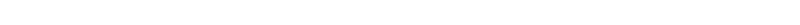And to calculate the nth root using logarithms, we simply need to divide these logs by n. Taking the inverse logarithm (exp) returns the answer.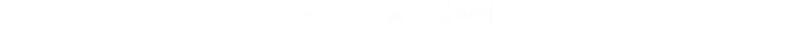### Example

Let's say we wanted to calculate the geometric mean of the following numbers: 4,9,16,69,42

 4 ln(4)=1.38629 9 ln(9)=2.19722 16 ln(16)=2.77259 69 ln(69)=4.23411 42 ln(42)=3.73767 TOTAL: 14.32788

14.32788 / 5 = 2.865577         exp( 2.865577 ) = 17.55918

The geometric mean of these five numbers is 17.55918

(You can confirm this by taking the fifth root of 4 × 9 × 16 × 69 × 42).

## Putting this into SQL

Converting this into SQL, we end up with the following statement. The logs are summed, this total is divided by the count, and finally this is used with exp function to invert the log.

select exp(sum(ln(column)) / count(*)) from data_table

There's a clever little additional optimisation that we can apply here. The sum divided by the count is the average, and we can replace the two aggregate functions with one. (Depending on your server this may, or may not, make a huge different to execution speed, but it certainly looks neater)

select exp(avg(ln(column))) from data_table

## Uses of Geometric Means

We've already seen how geometric means are useful when dealing with Compounding interest rates, what are other uses?

The arithmetic mean is relevant any time several quantities add together to produce a total. The arithmetic mean answers the question: “If all the quantities had the same value, what would that value have to be in order to achieve the same total?”

The geometric mean is relevant any time several quantities multiply together to produce a product. The geometric mean answers the question: “If all the quantities had the same value, what would that value have to be in order to achieve the same product?”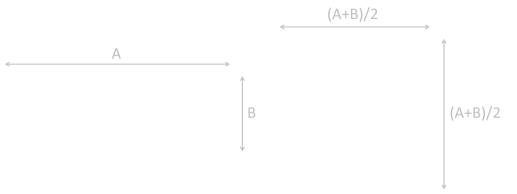An interesting way to picture the difference between the two means is to imagine a rectangle with sides length A and B. If the length of the perimeter of this rectangle were converted into a square, the length of the side of the square would be the arithmetic mean of the two sides. If these were fields, they would have the same length of fence surrounding them.
 If we were to create a square whose area is the same as the rectangle, then the length of the side of this square would be the geometric mean of the two sides of the rectangle. If these were fields, they would enclose the same area of land.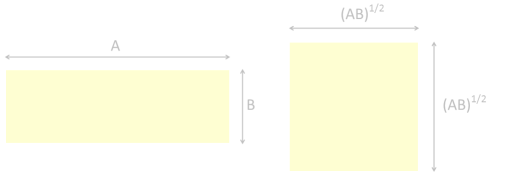The geometric mean is always less than (or equal) to the arithmetic mean. Specifically: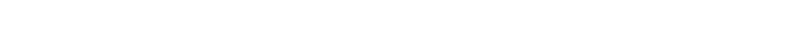You can see this, by squaring and rearranging. The inequality on the left, because it is squared, must always be greater than (or equal to) zero.### Screen Aspect Ratios

Have you ever wondered why the aspect ratios of widescreen format TV’s was selected at 16:9?

Before this ratio was formalized as a standard, there were a variety of formats, ranging for the original TV format 4:3 (1.33:1) to the very wide 2.35:1 CinemaScope.

Dr. Kerns H. Powers, a member of the SMPTE Working Group on High-Definition Electronic Production, first proposed the 16:9 (1.78:1) aspect ratio format as the best compromise between these two extremes.

Using paper silhouettes of the various formats and aligning their centers and scaling he was able to reach the compromise format, which we now know is the geometric mean of the two extreme ratios (all the other intermediate formats, naturally, fall between these two tallest and widest aspect ratio formats).Image Credit: MarkWarren (Wikipedia)

16:9 is the only widescreen aspect ratio natively supported by the DVD format.

You can find a complete list of all the articles here.Click here to receive email alerts on new articles.